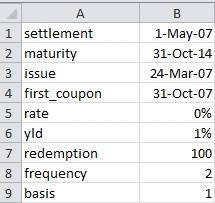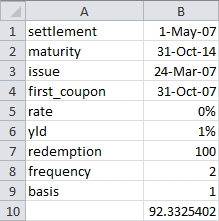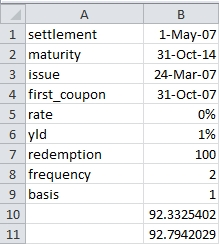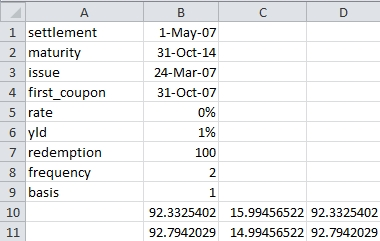## Correctly calculate the price of a bond with an odd long first period

Oct 18

Written by: Charles Flock
10/18/2011 8:12 PM11 Financial Calculations that you can’t do in EXCEL - Part 6 of 11
6. Correctly calculate the price of a bond with an odd long first period
Enter the following data into a worksheet.Enter the following formula in B10: =ODDFPRICE(B1,B2,B3,B4,B5,B6,B7,B8,B9)
This produces the following result.In this example, we have kept things very simple. The rate is zero, so the interest amount included in the calculation is zero. This bond has a long first coupon, with a quasi-coupon data on the 30th of April, 2007 and a first coupon on the 31st of October, 2007.
Since the settlement date is after the quasi-coupon date and since the rate is zero, then the PRICE function should return exactly the same answer as the ODDFPRICE function (for a more detailed explanation of the mechanics of this, you can read this article).
Enter the following formula in B11: =PRICE(B1,B2,B5,B6,B7,B8,B9)
This produces the following result.Quite clearly, the PRICE calculation and the ODDFPRICE calculation disagree, even though they use the same inputs and there is only one cash flow, the redemption amount.
Since we are using a zero-coupon bond, it is actually quite easy to see what the problem is. Enter the following formulae in the following cells:
C10         =LOG(B7/B10)/LOG(1+B6/B8)
C11         =LOG(B7/B11)/LOG(1+B6/B8)
D10        =((1+B6/B8)^-C10)*B7
D11        =((1+B6/B8)^-C11)*B7
This produces the following result.We have used EXCEL to tell us what value ODDFPRICE and PRICE used in determining the number of whole coupon plus the fractional coupon period until maturity and then double-checked that result. As you can see, they disagree by exactly one full coupon period. They can’t both be right.
Here’s what we get in SQL Server.
SELECT wct.ODDFPRICE(
'5/1/2007'       --settlement
,'10/31/2014'     --maturity
,'3/24/2007'      --issue
,'10/31/2007'     --first_coupon
,0.00             --rate
,.01              --yld
,100              --redemption
,2                --frequency
,1                --basis
)
This produces the following result
----------------------
92.7942029404091

(1 row(s) affected)

 Previous: Correctly calculate the accrued interest on a bond with an odd first period
 Next: Calculate the PRICE of a bond with a monthly coupon

Tags:
Categories:
Location: BlogsThe WestClinTech Blog

## Search Blogs

 KeywordsPhrase

## Blog Archives

Archive
 < December 2023 >
SunMonTueWedThuFriSat
262728293012
3456789
10111213141516
17181920212223
24252627282930
31123456
Monthly
Go

### SupportCopyright 2008-2023 Westclintech LLC         Privacy Policy        Terms of Service